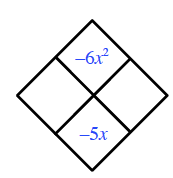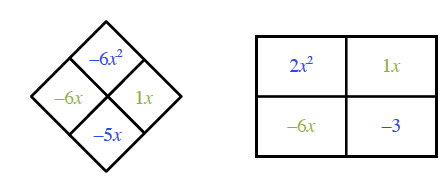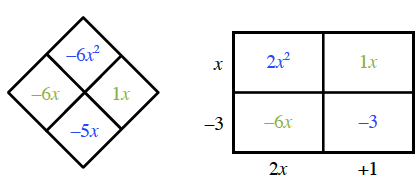### Home > CCA2 > Chapter Ch10 > Lesson 10.2.1 > Problem10-93

10-93.

Factor and simplify each expression below.

1. $\frac { x ^ { 2 } - 4 } { x ^ { 2 } + 4 x + 4 }$

2. $\frac { 2 x ^ { 2 } - 5 x - 3 } { 4 x ^ { 2 } + 4 x + 1 }$

3. Justify each step in simplifying the expression in part (a).

Factor and then reduce.

For the numerator, what numbers multiply to $-6$ and add to $-5?$ Diamond Pattern, top negative 6, x squared, bottom, negative 5, x.

Put these numbers in a generic rectangle (these create your "middle term") along with the first and last terms. Added to diamond problem, left, negative 6, x right, 1, x. 2 by 2 rectangle added, interior labeled as follows: top left, 2, x squared, top right, 1, x, bottom left, negative 6, x, bottom right, negative 3.
Now find the greatest common factors of each row and column.

See the generic rectangle. Added to rectangle, left edge, x minus 3, bottom edge, 2, x, + 1.
$2x^2 - 5x - 3 = (x - 3)(2x + 1)$

Now factor the denominator and simplify.